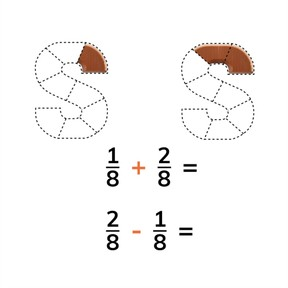Adding and subtracting fractions with common denominators

# Adding and subtracting fractions with common denominators

I can add and subtract simple fractions with common denominators.8,000 schools use Gynzy92,000 teachers use Gynzy1,600,000 students use Gynzy

## General

Students learn to add and subtract fractions with common denominators. They also learn to convert from fractions greater than 1 to mixed fractions.

## Common core standard(s)

CCSS.Math.Content.4.NF.B.3a

## Relevance

Discuss with students that it is useful to be able to add and subtract fractions with common denominators so you can determine the totals or differences of given fractions.

## Introduction

The students see a set of fractions on the interactive whiteboard and they must select which fraction to drag to match the given fractions.

## Development

Remind students that fractions with common denominators have denominators that are the same. Explain how to add fractions with common denominators with support of the visual. Remind students that they add the numerators and leave the denominators the same. Walk through a variety of problems that add fractions with common denominators. You can also choose to convert a whole number into a fraction. 1 is equal to 6//6. You can use this to make adding fractions easier. When you add fractions greater than 1, you can convert them into mixed fractions.
Show various ways of solving these addition problems- add to 1 and add on or convert whole numbers to fractions and then add. Have students practice with all varieties of adding fractions with common denominators. Then explain how to solve a fraction story problem and have them solve a few fraction word problems.
You can also subtract fractions with common denominators. You subtract the numerators and leave the denominators the same. Show students different subtraction problems in which they subtract different fractions with common denominators. When subtracting 1, you can convert the whole number into a fraction. 1 is equal to 7//7. You can use this to make your subtraction easier. You can also subtraction fractions from whole numbers. First make a whole fraction and determine the missing portion. If you end up with a fraction greater than 1, you can convert into a mixed fraction. Have students practice with a variety of subtraction problems to practice all of the subtraction methods. Finally explain how to solve a fraction story problem and have them solve a few fraction word problems.

Check that students understand adding and subtracting fractions with common denominators by asking the following questions:
- When do fractions have a common denominator?
- What do you do to add fractions with a common denominator?
- What do you do to subtract fractions with a common denominator?

## Guided practice

Students first practice adding fractions with a common denominator, then with subtraction, and then they are given fraction story problems.

## Closing

Discuss with students that it is important to be able to add and subtract fractions with common denominators, so you can determine the total of fractions or their difference. Ask students to determine which of the students on the interactive whiteboard has the correct answer. Ask students to explain their thinking. Then ask students to practice different addition and subtraction problems with fractions with common denominators.

## Teaching tips

Students who have difficulty with adding and subtracting fractions with common denominators can be supported by first gaining a better grasp of the parts of a fraction- the numerator and denominator ("down-ominator- goes on the bottom). Then have students match fractions that have common denominators. To add or subtract the fractions they might be helped with use of manipulatives.

### The online teaching platform for interactive whiteboards and displays in schools

• Save time building lessons

• Manage the classroom more efficiently

• Increase student engagement